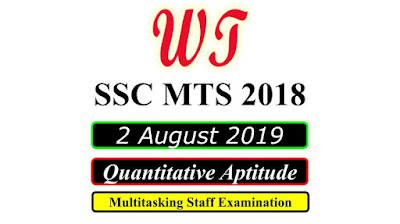Type Here to Get Search Results !

# [Download] SSC MTS 2 August 2019, Quantitative Questions PDF

Download SSC (Staff Selection Commission) MTS - Multi Tasking (Non-Technical) Staff Examination 2018 Tier 1 All Quantitative Aptitude Questions , Was Held on 19 August 2019 All Quantitative Aptitude Questions PDF With Answers.

Table of Content

Table of Content (toc)

## SSC MTS 2 August 2019 All Shifts Quantitative Questions PDF Download FreeSSC MTS 2 August 2019 All Shifts Quantitative Questions PDF Download Free

### SSC MTS 2 August 2019 Shift 1 Quantitative Questions

Instructions
For the following questions answer them individually
Q1:- A travels 15 km with a speed of 30 km/h. He travels another 25 km with a speed of 10 km/h. What is his average speed for the entire journey?
a.    40/3 km/h
b.    80/3 km/h
c.    20 km/h
d.    12 km/h
Q4:- What will be the compound interest on a sum of ₹ 1200 for 2 years at the rate of 20% per annum when the interest is compounded yearly?
a.    ₹ 624
b.    ₹ 504
c.    ₹ 576
d.    ₹ 528
Q5:- The simple interest on a sum fora certain number of years, same as the rate percentage of the interest, is equal to the sum itself. The number of years is equal to:
a.    5
b.    10
c.    8
d.    1
Q6:- Pipe A can fill a tank in 6 hours. Pipe B can fill the same tank in 8 hours. Pipe A, B and C together can fill the same tank in 12 hours.
Then which of the following statements is true for pipe C?
a.    It can fill the tank in 4 hours 40 minutes
b.    It can fill the tank in 4 hours 48 minutes
c.    It can empty the tank in 4 hours 48 minutes
d.    It can empty the tank in 4 hours 40 minutes
Q7:- By selling an article for ₹ 320, a man incurs a loss of 20%. What should be the selling price of an article to gain 20%?
a.    ₹ 450
b.    ₹ 480
c.    ₹ 420
d.    ₹ 500

### SSC MTS 2 August 2019 Shift 2 Quantitative Questions

Q2:- Aman spends 10% of his salary on food. He spends 20% of the remaining part on fuel. If he is left with ₹4680, then what is his salary?
a.    ₹5000
b.    ₹6000
c.    ₹5500
d.    ₹6500
Q3:- If after two years a sum becomes ₹4000 and after four years it becomes ₹6000 at the same rate   of compound interest (compounded annually), then what is the sum?
a.    ₹22888.88
b.    ₹2866.66
c.    ₹2666.66
d.    ₹22777.77
Q5:- if x+y+z=96 and x:y:z=3:4:5 then the value of z?
a.    32
b.    42
c.    36
d.    40
Q6:- Speed of a boat in still water is 8 km/h. If the speed of stream is 2 km/h, then how much distance can the boat travel in two hours going up stream?
a.    16 km
b.    20 km
c.    14 km
d.    12 km
Q7:- An article is sold for ₹1725 at 15% profit. If it is sold at 15% loss, then what will be the selling price?
a.    ₹1325
b.    ₹1275
c.    ₹1475
d.    ₹1025

### SSC MTS 2 August 2019 Shift 3 Quantitative Questions

Instructions
For the following questions answer them individually
Q1:- An article is sold for ₹ 2070 at a 15% profit. If the article is sold for ₹ 1890, then what will be the gain/loss percent?
a.    10% loss
b.    5% loss
c.    10% gain
d.    5%    gain
Q2:- What is the smallest number which when increased by 5 is divisible by 12, 18 and 30?
a.    175
b.    115
c.    235
d.    195
Q5:- A is 20% more than B, which is 25% less than C. Which of the following is true about A and C?
a.    A = 0.9C .
b.    A = 1.1C
c.    A = 0.95C
d.    A = C
Q6:- A mixture has milk and water in the ratio (by volume) of 8 : 3. If 3 litres of water is added to it, then new ratio of milk and water becomes 2 : 1. What are the quantities of milk and water respectively in the mixture initially?
a.    24 litres and 9 litres
b.    32 litres and 12 litres
c.    40 litres and 15 litres
d.    16 litres and 6 litres
Q7:- What is the average of first seven prime numbers (correct to two decimal places)?
a.    7.14
b.    8.76
c.    7.64
d.    8.29

SSC MTS Previous Year Paper

SSC MTS 2018

SSC MTS Reasoning Questions

SSC MTS Quantitative Questions

SSC MTS General English Questions

SSC MTS General Knowledge